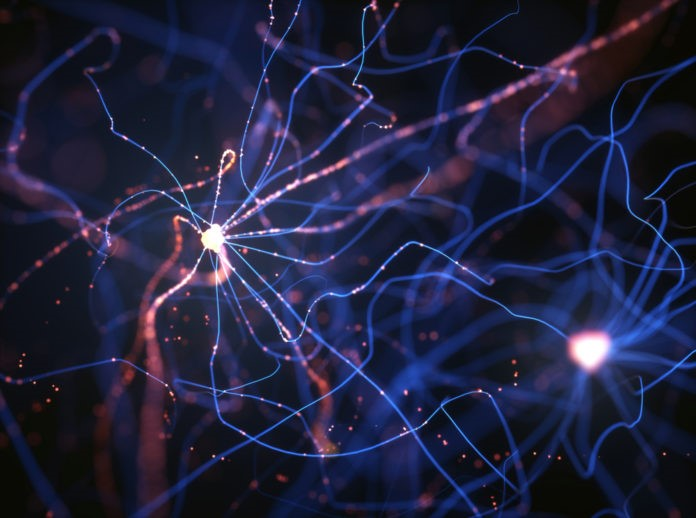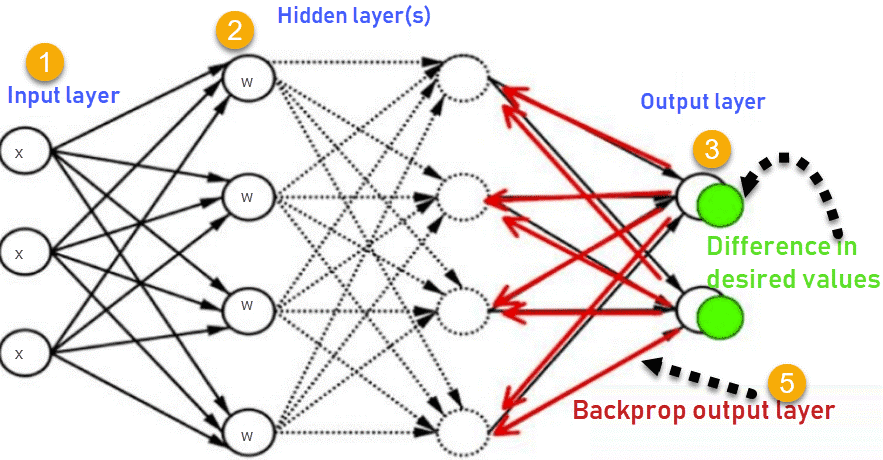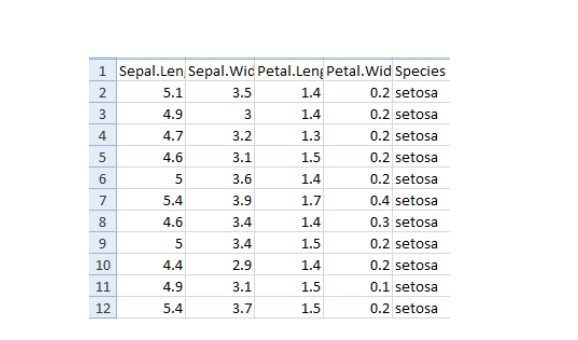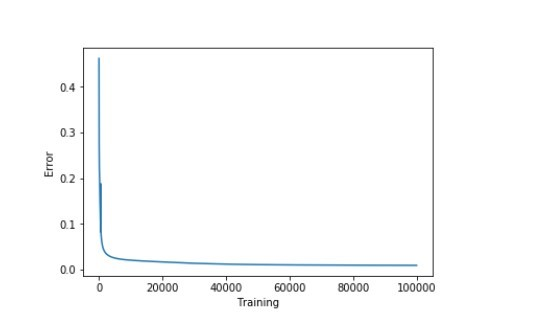# 反向傳播算法詳解1. 神經網絡
2. 什麼是反向傳播？
3. 反向傳播是如何工作的？
4. 損失函數
5. 為什麼我們需要反向傳播？
6. 前饋網絡
7. 反向傳播的類型
8. 案例研究

## 反向傳播是如何工作的？（How does backpropagation work?）

1.輸入層

2.隱藏層

3.輸出層1.輸入層接收x

2.使用權重w對輸入進行建模

3.每個隱藏層計算輸出，數據在輸出層準備就緒

4.實際輸出和期望輸出之間的差異稱為誤差

5.返回隱藏層並調整權重，以便在以後的運行中減少此錯誤

## 損失函數（Loss function）

•反向傳播快速、簡單且易於實現

•沒有要調整的參數

•不需要網絡的先驗知識，因此成為一種靈活的方法

•這種方法在大多數情況下都很有效

•模型不需要學習函數的特性

## 反向傳播的類型（Types of backpropagation）

•靜態反向傳播（Static backpropagation）

•循環反向傳播（Recurrent backpropagation）

1. 靜態反向傳播（Static backpropagation）

1. 循環反向傳播（Recurrent backpropagation）

## 案例研究（Case Study）

``import numpy as np  import pandas as pd  import seaborn as sns  import matplotlib.pyplot as plt  from sklearn.model_selection import train_test_split``

``import warnings  warnings.simplefilter(action='ignore', category=FutureWarning)``

``iris = pd.read_csv("iris.csv")  iris.head()````iris. replace (, , inplace=True)``

1.對輸出執行獨熱編碼（one hot encoding）。

2.執行sigmoid函數

3.標準化特徵

``def to_one_hot(Y):      n_col = np.amax(Y) + 1      binarized = np.zeros((len(Y), n_col))      for i in range(len(Y)):          binarized ] = 1.  return binarized``

``def sigmoid_func(x):      return 1/(1+np.exp(-x))  def sigmoid_derivative(x):      return sigmoid_func(x)*(1 – sigmoid_func(x))``

``def normalize (X, axis=-1, order=2):      l2 = np. atleast_1d (np.linalg.norm(X, order, axis))      l2 = 1  return X / np.expand_dims(l2, axis)``

``x = pd.DataFrame(iris, columns=columns)  x = normalize(x.as_matrix())    y = pd.DataFrame(iris, columns=columns)  y = y.as_matrix()  y = y.flatten()  y = to_one_hot(y)``

``#Split data to training and validation data（將數據拆分為訓練和驗證數據）  X_train, X_test, y_train, y_test = train_test_split(x, y, test_size=0.33)  #Weights  w0 = 2*np.random.random((4, 5)) - 1 #for input - 4 inputs, 3 outputs  w1 = 2*np.random.random((5, 3)) - 1 #for layer 1 - 5 inputs, 3 outputs  #learning rate  n = 0.1``

``errors = []``

``for i in range (100000):  #Feed forward network  layer0 = X_train  layer1 = sigmoid_func(np.dot(layer0, w0))  layer2 = sigmoid_func(np.dot(layer1, w1))  Back propagation using gradient descent  layer2_error = y_train - layer2  layer2_delta = layer2_error * sigmoid_derivative(layer2)  layer1_error = layer2_delta.dot (w1.T)  layer1_delta = layer1_error * sigmoid_derivative(layer1)  w1 += layer1.T.dot(layer2_delta) * n  w0 += layer0.T.dot(layer1_delta) * n  error = np.mean(np.abs(layer2_error))  errors.append(error)``

``accuracy_training = (1 - error) * 100``

``plt.plot(errors)  plt.xlabel('Training')  plt.ylabel('Error')  plt.show()````print ("Training Accuracy of the model " + str (round(accuracy_training,2)) + "%")``

Output: Training Accuracy of the model 99.04%

``#Validate  layer0 = X_test  layer1 = sigmoid_func(np.dot(layer0, w0))  layer2 = sigmoid_func(np.dot(layer1, w1))  layer2_error = y_test - layer2  error = np.mean(np.abs(layer2_error))  accuracy_validation = (1 - error) * 100  print ("Validation Accuracy of the model "+ str(round(accuracy_validation,2)) + "%")``

Output: Validation Accuracy 92.86%

•如果約束非常少，則系統可能不起作用

•過度訓練，過多的約束會導致過程緩慢

•只關注少數方面會導致偏見

•輸入數據是整體性能的關鍵

•有噪聲的數據會導致不準確的結果

•基於矩陣的方法優於小批量方法（mini-batch）